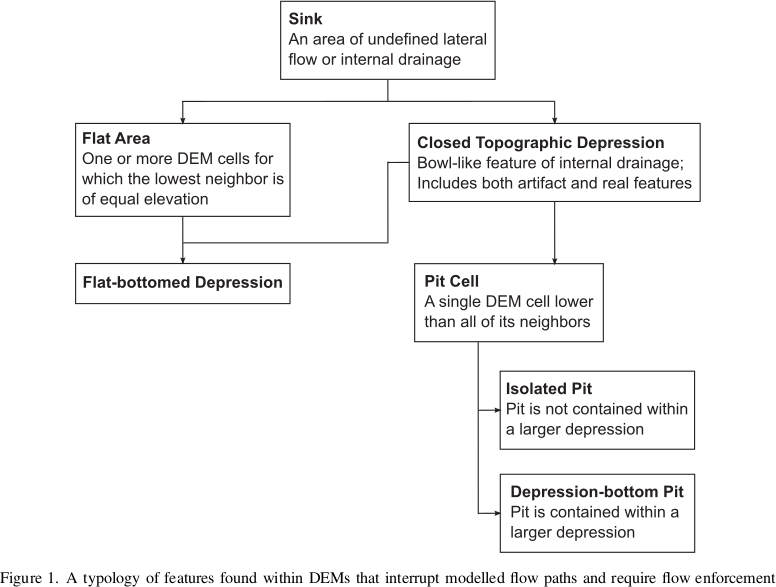# Depression-Filling¶

Depressions, otherwise known as pits, are areas of a landscape wherein flow ultimately terminates without reaching an ocean or the edge of a digital elevation model.

## Depressions, Pits, and Sinks¶

Depressions have been called by a variety of names. To clarify this mess, Lindsay (2016) provides a typology. This typology is followed here.## Original DEM¶

For reference, the original DEM appears as follows:

import richdem as rd
import numpy as np

beaufig = rd.rdShow(beau, ignore_colours=, axes=False, cmap='jet', figsize=(8,5.5))


## Complete Filling¶

Depression-filling is often used to fill in all the depressions in a DEM to the level of their lowest outlet or spill-point.

The result looks as follows:

beau_filled    = rd.FillDepressions(beau, in_place=False)
beaufig_filled = rd.rdShow(beau_filled, ignore_colours=, axes=False, cmap='jet', vmin=beaufig['vmin'], vmax=beaufig['vmax'], figsize=(8,5.5))


We can visualize the difference between the two like so:

beau_diff    = beau_filled - beau
beaufig_diff = rd.rdShow(beau_diff, ignore_colours=, axes=False, cmap='jet', figsize=(8,5.5))


Complete Filling is available via the following commands:

Language Command
Python richdem.FillDepressions
C++ richdem::FillDepression
 Pros Cons Fast Simple Leaves flat regions behind May modify large portions of a DEM

## Epsilon Filling¶

A downside of complete filling is that it replaces depressions with a perfectly flat region with no local gradients. One way to deal with this is to ensure that every cell in the region is raised some small amount, ε, above cells which are closer to a depression’s spill point.

This must be done carefully. In floating-point DEMs, the value ε is non-constant and must be chosen using the std::nextafter function. If a depression is too large, the imposed gradient may result in the interior of the depression being raised above the surrounding landscape. Using double instead of float reduces the potential for problems at a cost of twice the space used. If a problem does arise, RichDEM provides a warning.

We can visualize the difference between the epsilon-filled DEM and the original DEM like so:

beau_epsilon         = rd.FillDepressions(beau, epsilon=True, in_place=False)
beau_eps_diff        = beau_epsilon - beau
beaufig_eps_diff     = rd.rdShow(beau_eps_diff, ignore_colours=, axes=False, cmap='jet', figsize=(8,5.5))


We can visualize the difference between the epsilon-filled DEM and the completely-filled DEM as follows. Note that elevation increases with distance from the depression’s outlet: this is the effect of the epsilon.

beau_diffeps_diff    = beau_epsilon - beau_filled
beaufig_diffeps_diff = rd.rdShow(beau_diffeps_diff, ignore_colours=, axes=False, cmap='jet', figsize=(8,5.5))

Language Command
Python richdem.FillDepressions
C++ richdem::FillDepressionsEpsilon()
 Pros Cons All cells drain Not as fast as simple depression filling May modify large portions of a DEM May create elevated regions Success may depend on data type